# Rectangle pool

Determine dimensions of open pool with a square bottom with a capacity 32 m3 to have painted/bricked walls with least amount of material.

Result

a =  4 m
c =  2 m

#### Solution:

$V=32 \ \\ a=b \ \\ V=a^2 \ c \ \\ c=32/a^2 \ \\ S=a^2 +4ac=a^2 +4a (32/a^2) \ \\ S'=2a-128/a^2 \ \\ S'=0 \ \\ 2-128/a^3=0 \ \\ 1-64/a^3=0 \ \\ a^3=64 \ \\ a=\sqrt{ 64 }=4 \ \\ a=b=4 \ \text{m}$
$c=32/a^2=32/4^2=2 \ \text{m}$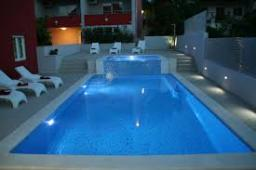Our examples were largely sent or created by pupils and students themselves. Therefore, we would be pleased if you could send us any errors you found, spelling mistakes, or rephasing the example. Thank you!

Leave us a comment of this math problem and its solution (i.e. if it is still somewhat unclear...):Be the first to comment!Tips to related online calculators
Looking for help with calculating roots of a quadratic equation?
Do you have a linear equation or system of equations and looking for its solution? Or do you have quadratic equation?
Tip: Our volume units converter will help you with the conversion of volume units.

#### You need to know the following knowledge to solve this word math problem:

We encourage you to watch this tutorial video on this math problem:

## Next similar math problems:

1. CarpetThe room is 10 x 5 meters. You have the role of carpet width of 1 meter. Make rectangular cut of roll that piece of carpet will be longest possible and it fit into the room. How long is a piece of carpet? Note .: carpet will not be parallel with the dia4 m long ladder touches the cube 1mx1m at the wall. How high reach on the wall?
3. Sphere in coneA sphere of radius 3 cm desribe cone with minimum volume. Determine cone dimensions.
4. Cone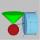Into rotating cone with dimensions r = 8 cm and h = 8 cm incribe cylinder with maximum volume so that the cylinder axis is perpendicular to the axis of the cone. Determine the dimensions of the cylinder.
5. Paper boxHard rectangular paper has dimensions of 60 cm and 28 cm. The corners are cut off equal squares and the residue was bent to form an open box. How long must be side of the squares to be the largest volume of the box?
6. Secret treasureScouts have a tent in the shape of a regular quadrilateral pyramid with a side of the base 4 m and a height of 3 m. Determine the radius r (and height h) of the container so that they can hide the largest possible treasure.
7. Alien shipThe alien ship has the shape of a sphere with a radius of r = 3000m, and its crew needs the ship to carry the collected research material in a cuboid box with a square base. Determine the length of the base and (and height h) so that the box has the large
8. Cylindrical containerAn open-topped cylindrical container has a volume of V = 3140 cm3. Find the cylinder dimensions (radius of base r, height v) so that the least material is needed to form the container.
9. Sphere and coneWithin the sphere of radius G = 33 cm inscribe cone with largest volume. What is that volume and what are the dimensions of the cone?
10. Goat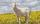Meadow is a circle with radius r = 19 m. How long must a rope to tie a goat to the pin on the perimeter of the meadow to allow goat eat half of meadow?
11. The positionThe position of a body at any time T is given by the displacement function S=t3-2t2-4t-8. Find its acceleration at each instant time when the velocity is zero.
12. Curve and lineThe equation of a curve C is y=2x² -8x+9 and the equation of a line L is x+ y=3 (1) Find the x co-ordinates of the points of intersection of L and C. (2) Show that one of these points is also the stationary point of C?
13. Minimum of sum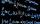Find a positive number that the sum of the number and its inverted value was minimal.
14. Derivation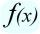Exists a function whose derivation is the same function?
15. Fall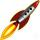The body was thrown vertically upward at speed v0 = 79 m/s. Body height versus time describe equation ?. What is the maximum height body reach?
16. Statue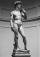On the pedestal high 4 m is statue 2.7 m high. At what distance from the statue must observer stand to see it in maximum viewing angle? Distance from the eye of the observer from the ground is 1.7 m.